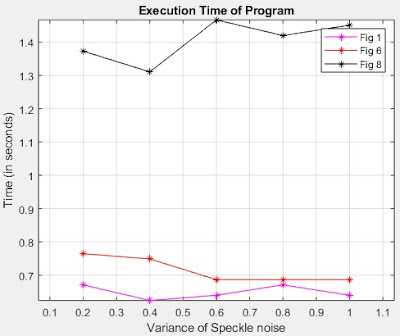Plotting Graph in Matlab | Matlab Code | Experimental Results Analysis. In any research paper, the "experimental results and discussion" section is one of the most important sections. It analyses the results and validates the proposed method. It is also helpful in performing the comparison among various methods. All this analysis can be performed by plotting various types of graphs. The line graph is one of the most popular graphs because of its simplicity and effectiveness. There are various tools using which you can plot the line graph like MS Excel etc. In comparison to other tools, the Matlab provides a more professional graph that looks much better for experimental results analysis. But in this post, we will learn to plot the line graph in Matlab.
x = [0.04 0.1 0.2 0.3 0.4 0.5 0.6];
y = [14.0342 10.1175 7.2147 5.5706 4.4740 3.7582 3.2600];
a = [4.9913 4.9287 4.7943 4.5631 4.2566 4.0485 3.9012];
b = [22.1004 18.1837 15.2809 13.6368 12.5402 11.8244 11.3262];
c = [13.0516 12.9950 12.8605 12.6293 12.3228 12.1147 11.9675];
plot(x, y, x, a, x, b, x, c)
Output
Matlab Code 2
x = [4 10 20 30 40 50 60];
y = [14.0342 10.1175 7.2147 5.5706 4.4740 3.7582 3.2600];
a = [4.9913 4.9287 4.7943 4.5631 4.2566 4.0485 3.9012];
plot(x, y, '-m*', x, a, '-r*')
xlabel('Speckle noise (in percentage)');
ylabel('SNR (in db)');
legend('Speckled Image', 'Log Transformed Speckled Image')
grid on, axis equal
title('Comparison Using Signal to Noise Ratio')
Output
Matlab Code 3
x = [0.2 0.4 0.6 0.8 1.0];
y = [0.6708 0.6240 0.6396 0.6708 0.6396];
a = [0.7644 0.7488 0.6864 0.6864 0.6864];
b = [1.3728 1.3104 1.4664 1.4196 1.4508];
plot(x, y, '-m*', x, a, '-r*', x, b, '-k*')
xlabel('Variance of Speckle noise');
ylabel('Time (in seconds)');
legend('Fig 1', 'Fig 6', 'Fig 8')
grid on, axis equal
title('Execution Time of Program')
OutputLine Graph 3

### Brief Explanation

The Matlab provides an in-built function i.e. plot(X,Y) that creates a 2-D line plot of the data in Y versus the corresponding values in X.

The Matlab provides an in-built function i.e. legend that creates a legend with descriptive labels for each plotted data series.

The Matlab provides an in-built function i.e. xlabel(txt) labels the x-axis of the current axes.

The Matlab provides an in-built function i.e. ylabel(txt) labels the y-axis of the current axes.

The Matlab provides an in-built function i.e. title(txt) that adds the specified title to the axes.

Different Types of SAR | Strip mapping Mode SAR | Spotlight Mode SAR | Inverse SAR (ISAR)

Problems in Synthetic Aperture Radar (SAR) Image

Plotting Graph in Matlab | Matlab Code | Experimental Results AnalysisReviewed by IPR on June 02, 2020 Rating: 5